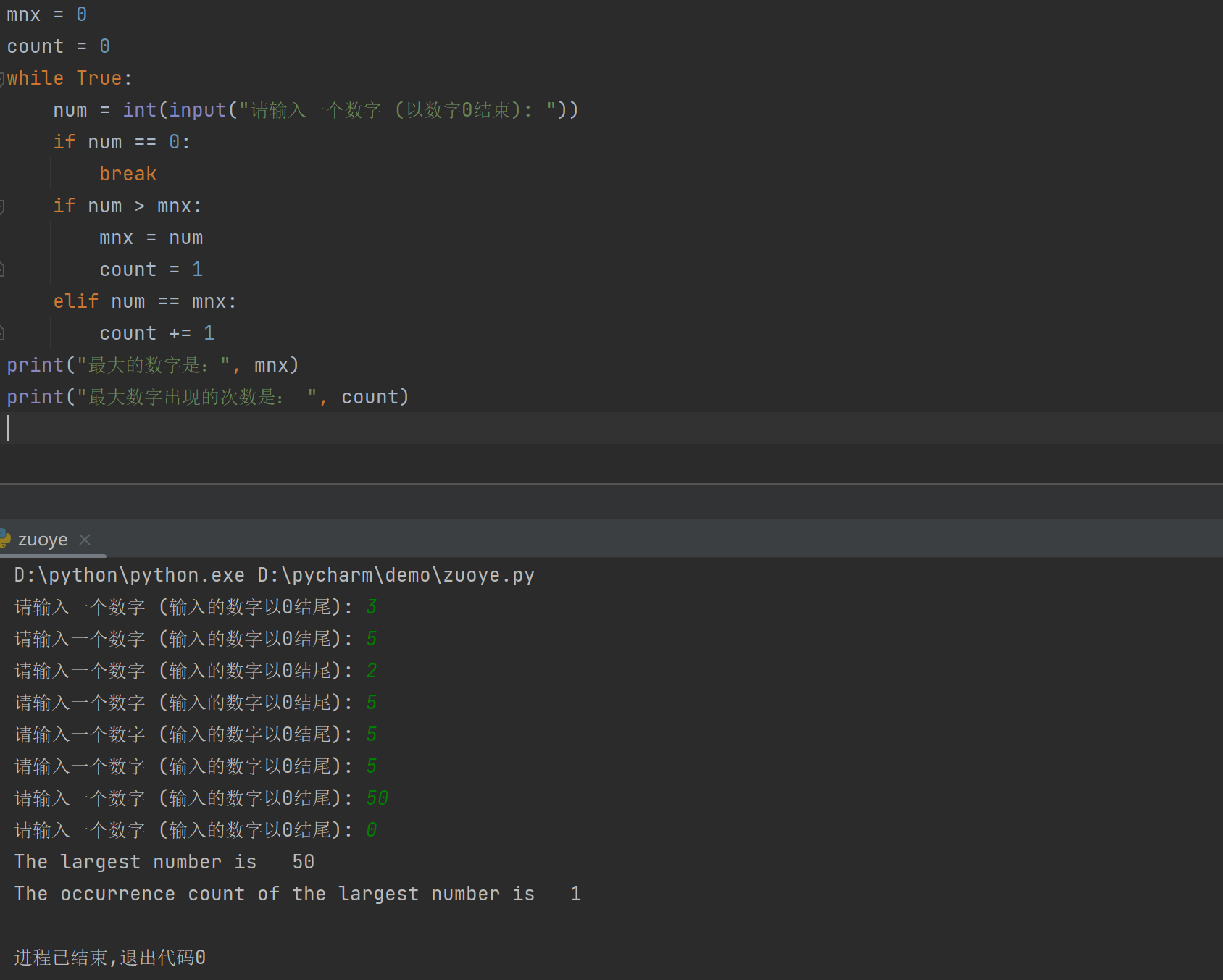## pyhton第九天作业

article/2023/9/24 21:11:08

(最大数的出现)编写程序读取整数，找出它们中的最大值，然后计算它的出现次数。假设输入以数字0 结束。假设你输入的是“3 5 2 5 5 50”;序找出的最大数是而 的出现次数是4(提示:维护两个变量 max和count。变量 max 存储的是当前最大数，而count 存储的是它的出现次数。初始状态下，将第一个值赋值给 max，将1赋值给 count。将 max 和每个随后的数字进行比较。如果这个数字大于 max，就将它赋值给 max 且将 ount重置为1。如果这个数等于max，给 count自增1。)

(蒙特卡罗模拟)一个正方形被分为四个更小的区域，如图a所示。如果你投掷一个飞镖到这个正方形一百万次，这个飞镖落在一个奇数区域里的概率是多少?编写程序模拟这个过程然后显式结果。(提示:将这个正方形的中心放在坐标系统的中心位置，如图b 所示。在正方形中随机产生一个点，然后统计这个点落入奇数区域的次数。

​编辑

​编辑

​编辑

​编辑

​编辑

MyTriangle 模块)创建一个名叫 MyTriangle 的模块，它包含下面两个函数

## (最大数的出现)编写程序读取整数，找出它们中的最大值，然后计算它的出现次数。 假设输入以数字0 结束。假设你输入的是“3 5 2 5 5 50”;序找出的最大数是而 的出现次数是4(提示:维护两个变量 max和count。变量 max 存储的是当前最大数，而count 存储的是它的出现次数。初始状态下，将第一个值赋值给 max，将1赋值给 count。将 max 和每个随后的数字进行比较。如果这个数字大于 max，就将它赋值给 max 且将 ount重置为1。如果这个数等于max，给 count自增1。)

``````mnx = 0
count = 0
while True:num = int(input("请输入一个数字 (以数字0结束): "))if num == 0:breakif num > mnx:mnx = numcount = 1elif num == mnx:count += 1
print("最大的数字是：", mnx)
print("最大数字出现的次数是： ", count)``````## (蒙特卡罗模拟)一个正方形被分为四个更小的区域，如图a所示。如果你投掷一个飞镖到这个正方形一百万次，这个飞镖落在一个奇数区域里的概率是多少?编写程序模拟这个过程然后显式结果。(提示:将这个正方形的中心放在坐标系统的中心位置，如图b 所示。在正方形中随机产生一个点，然后统计这个点落入奇数区域的次数。

``````
import random
sm = 0
for i in range(0, 1000001):x = random.random() * random.choice([-1, 1])y = random.random() * random.choice([-1, 1])if x <= 0 or (x >= 0 and y >= 0 and (y / (1 - x) >= 1)):sm += 1
a = sm / 1000000
print("1000000个随机点落在奇数区域的概率是%.12f" % a)
``````

### 求一个整数各个数字的和)编写一个函数，计算一个整数各个数字的和。使用下面的函数头:

``````number = int(input("请输入一个数字:"))def sumDigits(n):sm = 0while n != 0:sm += n % 10n = n // 10
``````

### 反向显示一个整数)编写下面的函数，反向显示一个整数

``````
number = int(input("请输入一个数字:"))def reverse(num):rum = 0while num != 0:rum = rum * 10 + num % 10num //= 10return rumprint(reverse(number))
``````

### 摄氏度和华氏度之间的转换)编写一个包含下面两个函数的模块

``````
def celsiusToFahrenheit(celsius):Fahrenheit = (9 / 5) * celsius + 32return Fahrenheitdef fahrenheitToCelsius(fahrenheit):celsius = (5 / 9) * (fahrenheit - 32)return celsiusprint("Celsius\t\tFahrenheit\t\tFahrenheit\tCelsius")
i = 40
for j in range(120, 20, -10):print(i, end="\t\t\t")print("%.1f" % (celsiusToFahrenheit(i)), end="\t\t\t")print(j, end="\t\t\t")print("%.2f" % (fahrenheitToCelsius(j)))i -= 1if i == 30:break``````

### 数列求和)编写一个函数计算下面的数列

``````
def m(i):sum = 0print(i, end="\t\t\t\t")for i in range(1, i + 1):sum += i / (i + 1)return sumprint("i\t\t\t\tm(i)")
for i in range(1, 21):print("%.4f" % m(i))``````

### MyTriangle 模块)创建一个名叫 MyTriangle 的模块，它包含下面两个函数

``````def isValid(side1, side2, side3):return side1 + side2 > side3 and side2 + side3 > side1 and side1 + side3 > side2def area(side1, side2, side3):s = (side1 + side2 + side3) / 2return (s * (s - side1) * (s - side2) * (s - side3)) ** 0.5s1, s2, s3 = eval(input("请输入三角形三边长:"))
if isValid(s1, s2, s3):print("area is %.2f" % area(s1, s2, s3))
else:print("Invalid")
``````### C++语法（11）---- 模拟实现list

1.基础元素 struct list_node {list_node* _next;list_node* _prev;T _data;list_node(const T& x): _next(nullptr), _prev(nullptr), _data(x){} }; list链表&#xff0c;基本要素就是链表的一个小块&#xff0c;这个小块自己带着的数据以及指向前后位置的指针组成。初始…

### TiDB K8S

1、 命名空间 k create ns ti k create namespace tidb-admin k create namespace tidb-clusteralias kkubectl alias tik -n tidb alias tiak -n tidb-admin alias tick -n tidb-cluster2、 Helm安装 tidb-operator helm repo add pingcap https://charts.pingcap.org/ helm…

### Netty进阶《ChannelPoolMap源码分析》

ChannelPoolMap是用来存储ChannelPool和指定key的一个集合Map&#xff0c;实际的应用场景就是服务器端是一个分布式集群服务&#xff0c;拥有多个配置地址&#xff0c;这样我们就可以配置多个服务地址&#xff0c;减轻单台服务器的压力&#xff1b;Netty框架提供了ChannelPoolM…

### 车机娱乐系统

BYD哪吒理想蔚来小鹏埃安吉利(魅族)大众 MQB大众MEB奥迪娱乐主机内置TboxYes生产商惠州比亚迪电子有限公司惠州市德赛西威汽车电子股份有限公司惠州市德赛西威汽车电子股份有限公司伟创力电脑&#xff08;苏州&#xff09;有限公司比亚迪汽车工业有限公司铁将军汽车电子股份有限…